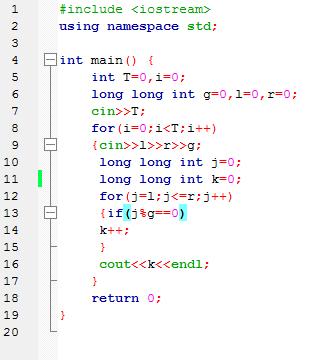This is the code in python for https://www.codechef.com/COOK106B/problems/GCDSET
import math
t=int(input())
while(t>0):
n=input().split()
l=int(n)
r=int(n)
g=int(n)
if(l>r):
print(0)
else:
if(g>r):
print(0)
if(g>=l and g<=r):
x=r//g
print(x)
if(g<l):
m=math.ceil(l/g)
y=r//g
if((y-m+1)==1):
print(0)
else:
print(y-m+1)
t=t-1

Check these test cases
6
3 4 3
6 7 3
2 10 3
3 10 3
2 9 3
3 9 3

1
0
3
3
3
3
Ping me if you need more test cases

1 Like

yeah…
6

3 4 3
1

6 7 3
0

2 10 3
3

3 10 3
3

2 9 3
3

3 9 3
3
i think the inbuilt ceil() of python is giving error…

1 LikeI can’t understand if i use a simple loop to calculate the answer…why it is saying “WRONG ANSWER”

check this one
1
6 7 3
0